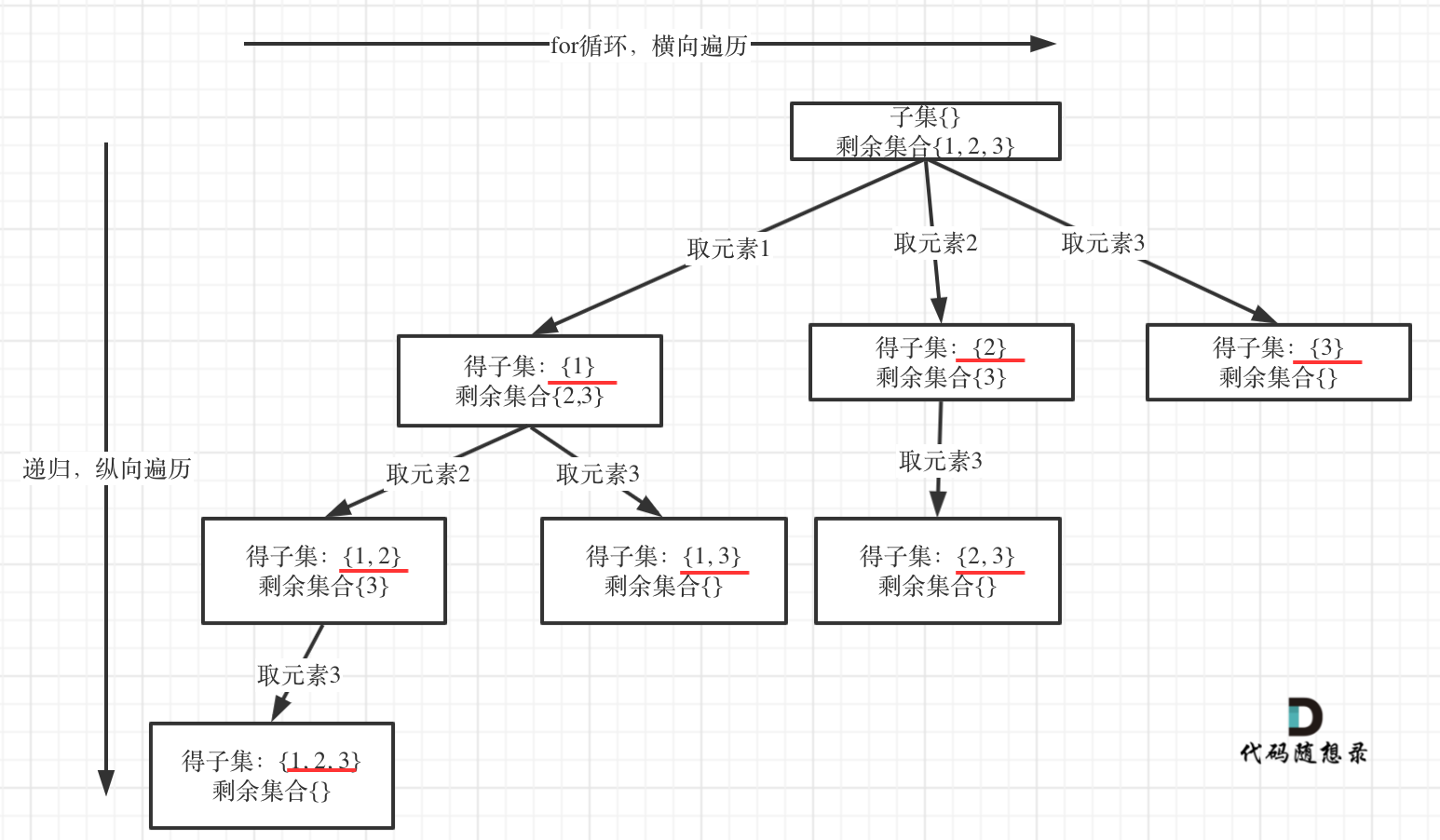## # 思路## # 回溯三部曲

• 递归函数参数

``````vector<vector<int>> result;
vector<int> path;
void backtracking(vector<int>& nums, int startIndex) {
``````
1
2
3
• 递归终止条件``````if (startIndex >= nums.size()) {
return;
}
``````
1
2
3

• 单层搜索逻辑

``````for (int i = startIndex; i < nums.size(); i++) {
path.push_back(nums[i]);    // 子集收集元素
backtracking(nums, i + 1);  // 注意从i+1开始，元素不重复取
path.pop_back();            // 回溯
}
``````
1
2
3
4
5

## # C++代码

``````void backtracking(参数) {
if (终止条件) {
存放结果;
return;
}

for (选择：本层集合中元素（树中节点孩子的数量就是集合的大小）) {
处理节点;
backtracking(路径，选择列表); // 递归
回溯，撤销处理结果
}
}
``````
1
2
3
4
5
6
7
8
9
10
11
12

``````class Solution {
private:
vector<vector<int>> result;
vector<int> path;
void backtracking(vector<int>& nums, int startIndex) {
result.push_back(path); // 收集子集，要放在终止添加的上面，否则会漏掉自己
if (startIndex >= nums.size()) { // 终止条件可以不加
return;
}
for (int i = startIndex; i < nums.size(); i++) {
path.push_back(nums[i]);
backtracking(nums, i + 1);
path.pop_back();
}
}
public:
vector<vector<int>> subsets(vector<int>& nums) {
result.clear();
path.clear();
backtracking(nums, 0);
return result;
}
};

``````
1
2
3
4
5
6
7
8
9
10
11
12
13
14
15
16
17
18
19
20
21
22
23
24

## # 其他语言版本

Java：

``````class Solution {
List<List<Integer>> result = new ArrayList<>();// 存放符合条件结果的集合
public List<List<Integer>> subsets(int[] nums) {
if (nums.length == 0){
return result;
}
subsetsHelper(nums, 0);
return result;
}

private void subsetsHelper(int[] nums, int startIndex){
if (startIndex >= nums.length){ //终止条件可不加
return;
}
for (int i = startIndex; i < nums.length; i++){
subsetsHelper(nums, i + 1);
path.removeLast();
}
}
}
``````
1
2
3
4
5
6
7
8
9
10
11
12
13
14
15
16
17
18
19
20
21
22
23
24

Python：

``````class Solution:
def subsets(self, nums: List[int]) -> List[List[int]]:
res = []
path = []
def backtrack(nums,startIndex):
res.append(path[:])  #收集子集，要放在终止添加的上面，否则会漏掉自己
for i in range(startIndex,len(nums)):  #当startIndex已经大于数组的长度了，就终止了，for循环本来也结束了，所以不需要终止条件
path.append(nums[i])
backtrack(nums,i+1)  #递归
path.pop()  #回溯
backtrack(nums,0)
return res
``````
1
2
3
4
5
6
7
8
9
10
11
12

Go：

``````var res [][]int
func subset(nums []int) [][]int {
res = make([][]int, 0)
sort.Ints(nums)
Dfs([]int{}, nums, 0)
return res
}
func Dfs(temp, nums []int, start int){
tmp := make([]int, len(temp))
copy(tmp, temp)
res = append(res, tmp)
for i := start; i < len(nums); i++{
//if i>start&&nums[i]==nums[i-1]{
//	continue
//}
temp = append(temp, nums[i])
Dfs(temp, nums, i+1)
temp = temp[:len(temp)-1]
}
}
``````
1
2
3
4
5
6
7
8
9
10
11
12
13
14
15
16
17
18
19
20

Javascript:

``````var subsets = function(nums) {
let result = []
let path = []
function backtracking(startIndex) {
result.push(path.slice())
for(let i = startIndex; i < nums.length; i++) {
path.push(nums[i])
backtracking(i + 1)
path.pop()
}
}
backtracking(0)
return result
};
``````
1
2
3
4
5
6
7
8
9
10
11
12
13
14